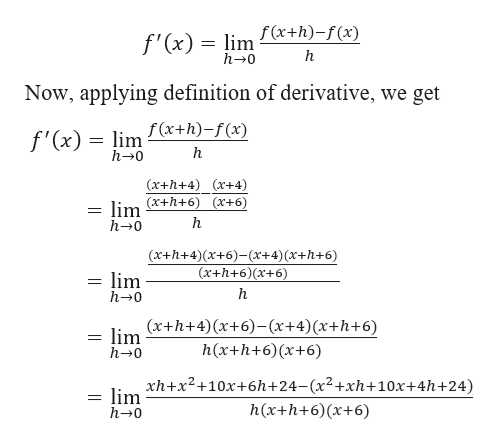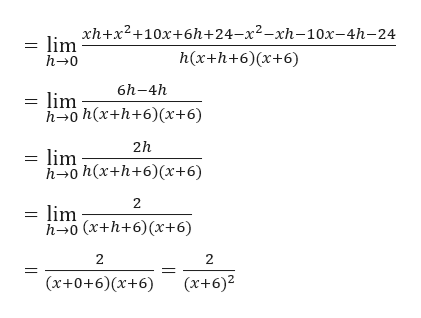# In this question you will use the definition of a derivative as a limit to find the derivative of f(x) = (x+4)/(x+6)f(x+h)-f(x)=?d/dx(x)=(I got 2/((x+6)^2) for this using the quotient rule

Question
1 views

In this question you will use the definition of a derivative as a limit to find the derivative of f(x) = (x+4)/(x+6)

f(x+h)-f(x)=?

d/dx(x)=

(I got 2/((x+6)^2) for this using the quotient rule

check_circle

Step 1

Given,

Step 2

Definition of derivative:help_outlineImage Transcriptionclosef(x+h)-f(x) f'(x) lim h h-0 Now, applying definition of derivative, we get lim x+h)-f(x) h 0 f'(x) (x+h+4) r+4) (x+h+6) (x+6) = lim h 0 (x+h+4)(x+6)-(x+4) (x+h+6) (x+h+6)(x+6) lim h 0 (x+h+4)(x+6)-(x+4)(x+h+6) lim h-0 h(x+h+6)x+6) xh+x2+10x+6h+24- (x2+xh+ 10x+4h+24) lim h 0 h(x+h+6) (x+6) fullscreen
Step 3

Further calcu...help_outlineImage Transcriptioncloseхh+x2+10х+6h+24-х2—хh-10х-4h-24 = lim h(x+h+6)(x+6) h- 0 6h-4h = lim h-о h(x+h+6)(x+6) 2h lim h-о h (x+h+6) (х+6) 2 lim h-0 (x+h+6)(x+6) 2 2 (x+0+6)(x+6) (x+6)2 fullscreen

### Want to see the full answer?

See Solution

#### Want to see this answer and more?

Solutions are written by subject experts who are available 24/7. Questions are typically answered within 1 hour.*

See Solution
*Response times may vary by subject and question.
Tagged in

### Derivative